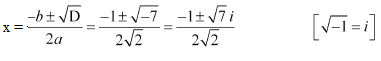# NCERT Solutions For Class 11 Math Chapter – 5 Exercise – 5.3

NCERT Solutions For Class 11 Math Chapter – 5 Exercise – 5.3

Solve each of the following equations:

1. x2 + 3 = 0

Solution:

x2 + 3 = 0

On comparing it with ax2 + bx + c = 0, we have

a = 1, b = 0, and c = 3

So, the discriminant of the given equation will be

D = b2 – 4ac = 02 – 4 × 1 × 3 = –12

Hence, the required solutions are: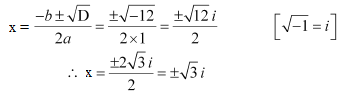2. 2x2 + x + 1 = 0

Solution:

2x2 + x + 1 = 0

On comparing it with ax2 + bx + c = 0, we have

a = 2, b = 1, and c =

1

So, the discriminant of the given equation will be

D = b2 – 4ac = 12 – 4 × 2 × 1 = 1 – 8 = –7

Hence, the required solutions are: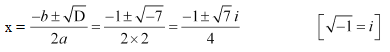3. x2 + 3x + 9 = 0

Solution:

x2 + 3x + 9 = 0

On comparing it with ax2 + bx + c = 0, we have

a = 1, b = 3, and c = 9

So, the discriminant of the given equation will be

D = b2 – 4ac = 32 – 4 × 1 × 9 = 9 – 36 = –27

Hence, the required solutions are: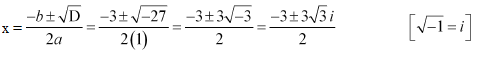4. –x2 + x – 2 = 0

Solution:

x2 + – 2 = 0

On comparing it with ax2 + bx + c = 0, we have

a = –1, b = 1, and c = –2

So, the discriminant of the given equation will be

D = b2 – 4ac = 12 – 4 × (–1) × (–2) = 1 – 8 = –7

Hence, the required solutions are: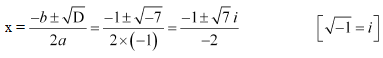5. x2 + 3x + 5 = 0

Solution:

x2 + 3x + 5 = 0

On comparing it with ax2 + bx + c = 0, we have

a = 1, b = 3, and c = 5

So, the discriminant of the given equation will be

D = b2 – 4ac = 32 – 4 × 1 × 5 =9 – 20 = –11

Hence, the required solutions are: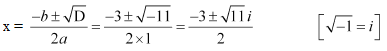6. x2 – x + 2 = 0

Solution:

x2 – x + 2 = 0

On comparing it with ax2 + bx + c = 0, we have

a = 1, b = –1, and c = 2

So, the discriminant of the given equation is

D = b2 – 4ac = (–1)2 – 4 × 1 × 2 = 1 – 8 = –7

Hence, the required solutions are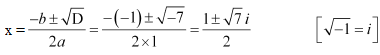7. √2x2 + x + √2 = 0

Solution:

√2x2 + x + √2 = 0

On comparing it with ax2 + bx + c = 0, we have

a = √2, b = 1, and c = √2

So, the discriminant of the given equation is

D = b2 – 4ac = (1)2 – 4 × √2 × √2 = 1 – 8 = –7

Hence, the required solutions are: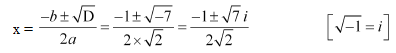8. √3x2 – √2x + 3√3 = 0

Solution:

√3x2 – √2x + 3√3 = 0

On comparing it with ax2 + bx + c = 0, we have

a = √3, b = -√2, and c = 3√3

So, the discriminant of the given equation is

D = b2 – 4ac = (-√2)2 – 4 × √3 × 3√3 = 2 – 36 = –34

Hence, the required solutions are: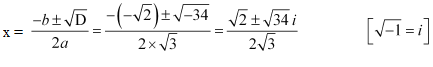9. x2 + x + 1/√2 = 0

Solution:

x2 + x + 1/√2 = 0

It can be rewritten as,

√2x2 + √2x + 1 = 0

On comparing it with ax2 + bx + c = 0, we have

a = √2, b = √2, and c = 1

So, the discriminant of the given equation is

D = b2 – 4ac = (√2)2 – 4 × √2 × 1 = 2 – 4√2 = 2(1 – 2√2)

Hence, the required solutions are: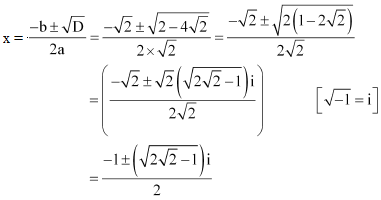10. x2 + x/√2 + 1 = 0

Solution:

x2 + x/√2 + 1 = 0

It can be rewritten as,

√2x2 + x + √2 = 0

On comparing it with ax2 + bx + c = 0, we have

a = √2, b = 1, and c = √2

So, the discriminant of the given equation is

D = b2 – 4ac = (1)2 – 4 × √2 × √2 = 1 – 8 = -7

Hence, the required solutions are: